# Mean State

Period Mean (original grids) [Watt m-2]
Model Period Mean (intersection) [Watt m-2]
Model Period Mean (complement) [Watt m-2]
Benchmark Period Mean (intersection) [Watt m-2]
Benchmark Period Mean (complement) [Watt m-2]
Bias [Watt m-2]
RMSE [Watt m-2]
Phase Shift [months]
Bias Score 
RMSE Score 
Seasonal Cycle Score 
Spatial Distribution Score 
Interannual Variability Score 
Overall Score 
Benchmark [-] 122.
CLM4 [-] 140. 140. 120. 164. 20.0 28.8 1.53 0.307 0.407 0.787 0.996 0.571 0.579
CLM4.5 [-] 136. 136. 120. 164. 16.2 27.0 1.56 0.370 0.399 0.779 0.988 0.578 0.585
CLM5 [-] 135. 135. 120. 164. 15.3 25.4 1.44 0.392 0.414 0.800 0.971 0.550 0.590
Period Mean (original grids) [Watt m-2]
Model Period Mean (intersection) [Watt m-2]
Model Period Mean (complement) [Watt m-2]
Benchmark Period Mean (intersection) [Watt m-2]
Benchmark Period Mean (complement) [Watt m-2]
Bias [Watt m-2]
RMSE [Watt m-2]
Phase Shift [months]
Bias Score 
RMSE Score 
Seasonal Cycle Score 
Spatial Distribution Score 
Interannual Variability Score 
Overall Score 
Benchmark [-] 102.
CLM4 [-] 113. 112. 101. 165. 11.6 25.8 1.39 0.587 0.446 0.801 0.988 0.658 0.654
CLM4.5 [-] 108. 108. 101. 165. 7.52 24.3 1.44 0.634 0.449 0.800 0.984 0.663 0.663
CLM5 [-] 108. 107. 101. 165. 6.68 23.4 1.50 0.648 0.461 0.780 0.991 0.617 0.660
Period Mean (original grids) [Watt m-2]
Model Period Mean (intersection) [Watt m-2]
Model Period Mean (complement) [Watt m-2]
Benchmark Period Mean (intersection) [Watt m-2]
Benchmark Period Mean (complement) [Watt m-2]
Bias [Watt m-2]
RMSE [Watt m-2]
Phase Shift [months]
Bias Score 
RMSE Score 
Seasonal Cycle Score 
Spatial Distribution Score 
Interannual Variability Score 
Overall Score 
Benchmark [-] 112.
CLM4 [-] 117. 117. 110. 159. 8.28 23.2 1.11 0.627 0.557 0.850 0.974 0.576 0.690
CLM4.5 [-] 112. 111. 110. 159. 3.69 22.5 1.11 0.654 0.555 0.851 0.948 0.592 0.692
CLM5 [-] 113. 113. 110. 159. 5.35 22.1 1.18 0.665 0.561 0.838 0.962 0.555 0.690
Period Mean (original grids) [Watt m-2]
Model Period Mean (intersection) [Watt m-2]
Model Period Mean (complement) [Watt m-2]
Benchmark Period Mean (intersection) [Watt m-2]
Benchmark Period Mean (complement) [Watt m-2]
Bias [Watt m-2]
RMSE [Watt m-2]
Phase Shift [months]
Bias Score 
RMSE Score 
Seasonal Cycle Score 
Spatial Distribution Score 
Interannual Variability Score 
Overall Score 
Benchmark [-] 43.3
CLM4 [-] 13.9 14.0 43.2 44.3 -29.8 36.0 0.644 0.587 0.661 0.957 0.897 0.677 0.740
CLM4.5 [-] 18.2 18.3 43.2 44.3 -26.3 31.3 0.427 0.629 0.701 0.972 0.782 0.643 0.738
CLM5 [-] 14.6 14.7 43.2 44.3 -29.9 33.5 0.368 0.589 0.703 0.976 0.758 0.638 0.728
Period Mean (original grids) [Watt m-2]
Model Period Mean (intersection) [Watt m-2]
Model Period Mean (complement) [Watt m-2]
Benchmark Period Mean (intersection) [Watt m-2]
Benchmark Period Mean (complement) [Watt m-2]
Bias [Watt m-2]
RMSE [Watt m-2]
Phase Shift [months]
Bias Score 
RMSE Score 
Seasonal Cycle Score 
Spatial Distribution Score 
Interannual Variability Score 
Overall Score 
Benchmark [-] 144.
CLM4 [-] 163. 163. 140. 160. 25.9 34.8 1.68 0.304 0.390 0.753 0.830 0.496 0.527
CLM4.5 [-] 162. 161. 140. 160. 24.8 33.9 1.65 0.314 0.391 0.757 0.856 0.491 0.533
CLM5 [-] 157. 156. 140. 160. 20.0 30.5 1.51 0.352 0.394 0.784 0.838 0.478 0.540
Period Mean (original grids) [Watt m-2]
Model Period Mean (intersection) [Watt m-2]
Model Period Mean (complement) [Watt m-2]
Benchmark Period Mean (intersection) [Watt m-2]
Benchmark Period Mean (complement) [Watt m-2]
Bias [Watt m-2]
RMSE [Watt m-2]
Phase Shift [months]
Bias Score 
RMSE Score 
Seasonal Cycle Score 
Spatial Distribution Score 
Interannual Variability Score 
Overall Score 
Benchmark [-] 62.5
CLM4 [-] 48.7 48.6 62.3 75.7 -13.6 27.3 0.293 0.790 0.672 0.980 0.763 0.651 0.755
CLM4.5 [-] 46.3 46.3 62.3 75.7 -15.9 29.4 0.271 0.765 0.662 0.981 0.702 0.656 0.738
CLM5 [-] 42.4 42.4 62.3 75.7 -19.8 31.9 0.344 0.710 0.651 0.976 0.876 0.660 0.754
Period Mean (original grids) [Watt m-2]
Model Period Mean (intersection) [Watt m-2]
Model Period Mean (complement) [Watt m-2]
Benchmark Period Mean (intersection) [Watt m-2]
Benchmark Period Mean (complement) [Watt m-2]
Bias [Watt m-2]
RMSE [Watt m-2]
Phase Shift [months]
Bias Score 
RMSE Score 
Seasonal Cycle Score 
Spatial Distribution Score 
Interannual Variability Score 
Overall Score 
Benchmark [-] 72.7
CLM4 [-] 70.4 70.2 72.1 94.9 -0.664 29.1 0.706 0.709 0.592 0.932 0.813 0.633 0.712
CLM4.5 [-] 68.9 68.7 72.1 94.9 -2.05 29.8 0.698 0.699 0.588 0.933 0.821 0.632 0.710
CLM5 [-] 66.3 66.0 72.1 94.9 -4.76 30.0 0.732 0.713 0.572 0.930 0.889 0.643 0.720
Period Mean (original grids) [Watt m-2]
Model Period Mean (intersection) [Watt m-2]
Model Period Mean (complement) [Watt m-2]
Benchmark Period Mean (intersection) [Watt m-2]
Benchmark Period Mean (complement) [Watt m-2]
Bias [Watt m-2]
RMSE [Watt m-2]
Phase Shift [months]
Bias Score 
RMSE Score 
Seasonal Cycle Score 
Spatial Distribution Score 
Interannual Variability Score 
Overall Score 
Benchmark [-] 109.
CLM4 [-] 126. 126. 105. 157. 24.5 37.0 0.934 0.407 0.486 0.892 0.992 0.578 0.640
CLM4.5 [-] 122. 122. 105. 157. 21.4 34.9 0.926 0.450 0.485 0.893 0.992 0.565 0.645
CLM5 [-] 121. 121. 105. 157. 20.0 33.3 0.825 0.463 0.500 0.916 0.990 0.522 0.649
Period Mean (original grids) [Watt m-2]
Model Period Mean (intersection) [Watt m-2]
Model Period Mean (complement) [Watt m-2]
Benchmark Period Mean (intersection) [Watt m-2]
Benchmark Period Mean (complement) [Watt m-2]
Bias [Watt m-2]
RMSE [Watt m-2]
Phase Shift [months]
Bias Score 
RMSE Score 
Seasonal Cycle Score 
Spatial Distribution Score 
Interannual Variability Score 
Overall Score 
Benchmark [-] 41.8
CLM4 [-] 11.0 11.4 41.9 41.5 -31.3 34.0 0.385 0.555 0.671 0.975 0.794 0.703 0.728
CLM4.5 [-] 12.6 13.0 41.9 41.5 -30.0 33.0 0.404 0.574 0.669 0.973 0.715 0.698 0.716
CLM5 [-] 9.84 10.2 41.9 41.5 -32.9 33.8 0.382 0.541 0.671 0.975 0.711 0.702 0.712
Period Mean (original grids) [Watt m-2]
Model Period Mean (intersection) [Watt m-2]
Model Period Mean (complement) [Watt m-2]
Benchmark Period Mean (intersection) [Watt m-2]
Benchmark Period Mean (complement) [Watt m-2]
Bias [Watt m-2]
RMSE [Watt m-2]
Phase Shift [months]
Bias Score 
RMSE Score 
Seasonal Cycle Score 
Spatial Distribution Score 
Interannual Variability Score 
Overall Score 
Benchmark [-] 96.5
CLM4 [-] 98.7 98.7 93.2 138. 6.87 22.9 0.598 0.715 0.649 0.952 0.916 0.592 0.746
CLM4.5 [-] 98.5 98.4 93.2 138. 6.64 22.9 0.621 0.720 0.647 0.948 0.899 0.603 0.744
CLM5 [-] 97.5 97.5 93.2 138. 5.79 23.2 0.580 0.720 0.643 0.954 0.928 0.604 0.749
Period Mean (original grids) [Watt m-2]
Model Period Mean (intersection) [Watt m-2]
Model Period Mean (complement) [Watt m-2]
Benchmark Period Mean (intersection) [Watt m-2]
Benchmark Period Mean (complement) [Watt m-2]
Bias [Watt m-2]
RMSE [Watt m-2]
Phase Shift [months]
Bias Score 
RMSE Score 
Seasonal Cycle Score 
Spatial Distribution Score 
Interannual Variability Score 
Overall Score 
Benchmark [-] 64.4
CLM4 [-] 64.0 64.0 61.9 90.5 2.72 25.3 0.317 0.782 0.687 0.979 0.841 0.760 0.789
CLM4.5 [-] 65.5 65.5 61.9 90.5 4.14 25.4 0.308 0.788 0.681 0.980 0.888 0.766 0.797
CLM5 [-] 60.9 61.0 61.9 90.5 -0.150 25.5 0.326 0.810 0.671 0.978 0.880 0.736 0.791
Period Mean (original grids) [Watt m-2]
Model Period Mean (intersection) [Watt m-2]
Model Period Mean (complement) [Watt m-2]
Benchmark Period Mean (intersection) [Watt m-2]
Benchmark Period Mean (complement) [Watt m-2]
Bias [Watt m-2]
RMSE [Watt m-2]
Phase Shift [months]
Bias Score 
RMSE Score 
Seasonal Cycle Score 
Spatial Distribution Score 
Interannual Variability Score 
Overall Score 
Benchmark [-] 115.
CLM4 [-] 74.6 74.6 77.4 133. -0.847 29.1 0.799 0.585 0.565 0.907 0.865 0.574 0.677
CLM4.5 [-] 73.7 73.7 77.4 133. -1.61 28.6 0.797 0.599 0.565 0.907 0.883 0.575 0.682
CLM5 [-] 71.5 71.5 77.4 133. -4.18 29.2 0.799 0.588 0.564 0.907 0.864 0.564 0.675
Period Mean (original grids) [Watt m-2]
Model Period Mean (intersection) [Watt m-2]
Model Period Mean (complement) [Watt m-2]
Benchmark Period Mean (intersection) [Watt m-2]
Benchmark Period Mean (complement) [Watt m-2]
Bias [Watt m-2]
RMSE [Watt m-2]
Phase Shift [months]
Bias Score 
RMSE Score 
Seasonal Cycle Score 
Spatial Distribution Score 
Interannual Variability Score 
Overall Score 
Benchmark [-] 54.9
CLM4 [-] 64.2 64.2 51.3 143. 15.0 32.5 1.48 0.524 0.476 0.800 0.854 0.495 0.604
CLM4.5 [-] 64.8 64.8 51.3 143. 15.7 32.9 1.51 0.517 0.473 0.797 0.865 0.498 0.604
CLM5 [-] 64.5 64.6 51.3 143. 15.6 32.9 1.47 0.522 0.471 0.802 0.878 0.502 0.608
Period Mean (original grids) [Watt m-2]
Model Period Mean (intersection) [Watt m-2]
Model Period Mean (complement) [Watt m-2]
Benchmark Period Mean (intersection) [Watt m-2]
Benchmark Period Mean (complement) [Watt m-2]
Bias [Watt m-2]
RMSE [Watt m-2]
Phase Shift [months]
Bias Score 
RMSE Score 
Seasonal Cycle Score 
Spatial Distribution Score 
Interannual Variability Score 
Overall Score 
Benchmark [-] 44.9
CLM4 [-] 19.2 19.0 44.8 45.6 -27.4 32.4 0.234 0.615 0.695 0.984 0.805 0.654 0.741
CLM4.5 [-] 20.5 20.3 44.8 45.6 -26.3 31.1 0.217 0.627 0.702 0.985 0.788 0.634 0.740
CLM5 [-] 15.2 15.0 44.8 45.6 -31.6 35.1 0.181 0.569 0.700 0.988 0.751 0.638 0.724
Period Mean (original grids) [Watt m-2]
Model Period Mean (intersection) [Watt m-2]
Model Period Mean (complement) [Watt m-2]
Benchmark Period Mean (intersection) [Watt m-2]
Benchmark Period Mean (complement) [Watt m-2]
Bias [Watt m-2]
RMSE [Watt m-2]
Phase Shift [months]
Bias Score 
RMSE Score 
Seasonal Cycle Score 
Spatial Distribution Score 
Interannual Variability Score 
Overall Score 
Benchmark [-] 134.
CLM4 [-] 117. 117. 127. 169. -2.29 26.7 1.12 0.589 0.576 0.860 0.969 0.600 0.695
CLM4.5 [-] 118. 118. 127. 169. -0.859 28.6 1.16 0.554 0.571 0.856 0.938 0.608 0.683
CLM5 [-] 116. 116. 127. 169. -2.92 27.2 1.04 0.567 0.585 0.876 0.956 0.572 0.690
Period Mean (original grids) [Watt m-2]
Model Period Mean (intersection) [Watt m-2]
Model Period Mean (complement) [Watt m-2]
Benchmark Period Mean (intersection) [Watt m-2]
Benchmark Period Mean (complement) [Watt m-2]
Bias [Watt m-2]
RMSE [Watt m-2]
Phase Shift [months]
Bias Score 
RMSE Score 
Seasonal Cycle Score 
Spatial Distribution Score 
Interannual Variability Score 
Overall Score 
Benchmark [-] 99.6
CLM4 [-] 98.2 98.6 97.8 136. 2.49 24.7 0.466 0.814 0.640 0.965 0.809 0.617 0.748
CLM4.5 [-] 96.1 96.5 97.8 136. 0.486 24.8 0.425 0.815 0.640 0.968 0.793 0.624 0.747
CLM5 [-] 89.4 89.7 97.8 136. -6.20 25.6 0.491 0.810 0.631 0.964 0.811 0.585 0.739
Period Mean (original grids) [Watt m-2]
Model Period Mean (intersection) [Watt m-2]
Model Period Mean (complement) [Watt m-2]
Benchmark Period Mean (intersection) [Watt m-2]
Benchmark Period Mean (complement) [Watt m-2]
Bias [Watt m-2]
RMSE [Watt m-2]
Phase Shift [months]
Bias Score 
RMSE Score 
Seasonal Cycle Score 
Spatial Distribution Score 
Interannual Variability Score 
Overall Score 
Benchmark [-] 72.2
CLM4 [-] 61.3 61.5 72.0 77.2 -10.8 24.9 0.383 0.827 0.687 0.973 0.876 0.613 0.777
CLM4.5 [-] 61.5 61.6 72.0 77.2 -10.6 24.4 0.391 0.829 0.690 0.973 0.931 0.607 0.786
CLM5 [-] 54.7 54.9 72.0 77.2 -17.2 29.2 0.370 0.739 0.664 0.974 0.957 0.615 0.769
Period Mean (original grids) [Watt m-2]
Model Period Mean (intersection) [Watt m-2]
Model Period Mean (complement) [Watt m-2]
Benchmark Period Mean (intersection) [Watt m-2]
Benchmark Period Mean (complement) [Watt m-2]
Bias [Watt m-2]
RMSE [Watt m-2]
Phase Shift [months]
Bias Score 
RMSE Score 
Seasonal Cycle Score 
Spatial Distribution Score 
Interannual Variability Score 
Overall Score 
Benchmark [-] 81.6
CLM4 [-] 68.2 68.7 81.1 116. -11.7 23.0 0.511 0.748 0.693 0.960 0.981 0.638 0.786
CLM4.5 [-] 66.3 66.8 81.1 116. -13.5 24.3 0.497 0.726 0.690 0.961 0.973 0.636 0.779
CLM5 [-] 64.3 64.9 81.1 116. -15.5 26.1 0.532 0.704 0.677 0.959 0.986 0.634 0.773
Period Mean (original grids) [Watt m-2]
Model Period Mean (intersection) [Watt m-2]
Model Period Mean (complement) [Watt m-2]
Benchmark Period Mean (intersection) [Watt m-2]
Benchmark Period Mean (complement) [Watt m-2]
Bias [Watt m-2]
RMSE [Watt m-2]
Phase Shift [months]
Bias Score 
RMSE Score 
Seasonal Cycle Score 
Spatial Distribution Score 
Interannual Variability Score 
Overall Score 
Benchmark [-] 132.
CLM4 [-] 154. 154. 131. 178. 24.1 30.9 1.10 0.275 0.412 0.850 0.898 0.516 0.561
CLM4.5 [-] 152. 152. 131. 178. 21.7 29.1 1.11 0.311 0.410 0.846 0.936 0.519 0.572
CLM5 [-] 151. 151. 131. 178. 20.5 27.7 1.16 0.327 0.416 0.840 0.919 0.508 0.571
Period Mean (original grids) [Watt m-2]
Model Period Mean (intersection) [Watt m-2]
Model Period Mean (complement) [Watt m-2]
Benchmark Period Mean (intersection) [Watt m-2]
Benchmark Period Mean (complement) [Watt m-2]
Bias [Watt m-2]
RMSE [Watt m-2]
Phase Shift [months]
Bias Score 
RMSE Score 
Seasonal Cycle Score 
Spatial Distribution Score 
Interannual Variability Score 
Overall Score 
Benchmark [-] 117.
CLM4 [-] 127. 126. 117. 145. 10.3 24.7 0.910 0.628 0.535 0.892 0.935 0.589 0.686
CLM4.5 [-] 123. 123. 117. 145. 7.33 23.1 0.870 0.691 0.537 0.901 0.964 0.603 0.706
CLM5 [-] 121. 121. 117. 145. 5.09 21.7 1.01 0.712 0.547 0.879 0.926 0.548 0.693
Period Mean (original grids) [Watt m-2]
Model Period Mean (intersection) [Watt m-2]
Model Period Mean (complement) [Watt m-2]
Benchmark Period Mean (intersection) [Watt m-2]
Benchmark Period Mean (complement) [Watt m-2]
Bias [Watt m-2]
RMSE [Watt m-2]
Phase Shift [months]
Bias Score 
RMSE Score 
Seasonal Cycle Score 
Spatial Distribution Score 
Interannual Variability Score 
Overall Score 
Benchmark [-] 95.7
CLM4 [-] 84.1 83.8 92.5 124. -8.67 26.8 0.560 0.724 0.661 0.951 0.937 0.522 0.743
CLM4.5 [-] 82.4 82.0 92.5 124. -10.2 27.7 0.601 0.709 0.659 0.944 0.917 0.525 0.735
CLM5 [-] 81.9 81.5 92.5 124. -10.7 27.6 0.604 0.713 0.660 0.943 0.907 0.521 0.734

# Temporally integrated period mean

BENCHMARK MEAN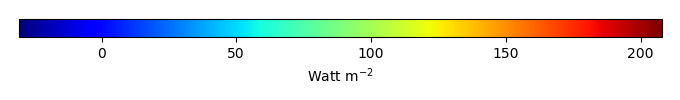MODEL MEANBIAS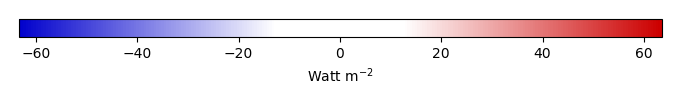BIAS SCORERMSE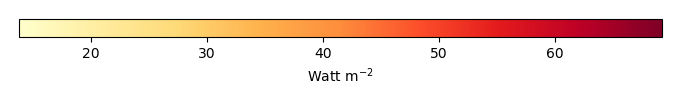RMSE SCOREBENCHMARK INTERANNUAL VARIABILITY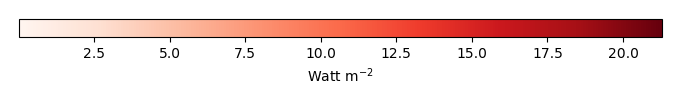MODEL INTERANNUAL VARIABILITYINTERANNUAL VARIABILITY SCOREBENCHMARK MAX MONTHMODEL MAX MONTHDIFFERENCE IN MAX MONTH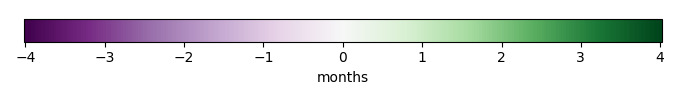SEASONAL CYCLE SCORESPATIAL TAYLOR DIAGRAMMODEL COLORS# Spatially integrated regional mean

MODEL COLORSREGIONAL MEANANNUAL CYCLEMONTHLY ANOMALYANNUAL CYCLE# All Models

BenchmarkCLM4CLM4.5CLM5# Data Information

creation_date: Tue Jul 1 08:16:58 PDT 2014

source_file: This product is generated from monthly 1 degree GEWEX SRB Radiation observations